# How to create hidden questions (sys_range)

Quite often it is useful to create hidden questions variables, that can have the values stored in the data set and can be used for further logic or piping. In this we will use the system variable "sys_range". This is a very useful system variable that will limit the allowed answers to those allowed by answer control. Combined with the autofill function, this will create hidden questions and dummy questions quite easily.

An practical example: Segmentation
Based on a 4 row grid question, we want to calculate the respondents score in-line, and use the respondent's segment belonging in the rest of the questionnaire

1. The origin is a normal grid question
The grid question looks like this in the editor (Note that in this case the code direction has been set to descending, since the high scores = positive comes first)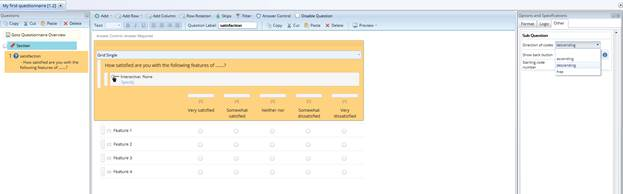On the web the question looks like this: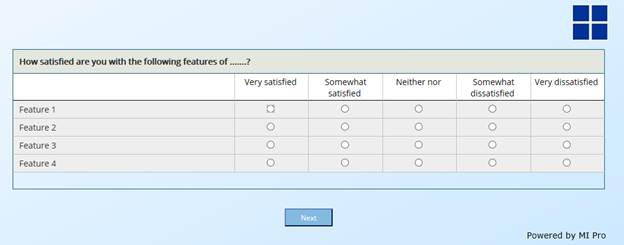2. Calculating the index score and segment belonging
We now want to calculate the index-scores. We will now use the sum 2 function, adding together the scores from the grid.

The segments are defined as follows:

• Super positive = Score 19-20
• Positive = Score 15 – 18
• Neutral = Score 10 – 14
• Negative = Less than 10

The scores in this example are as follows: 5,2,2 and 4 i.e. a sum of 13. The respondent therefore belong to the "Neutral"-segment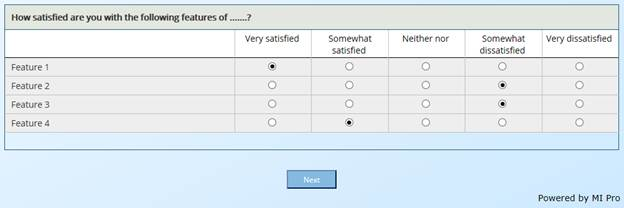The first question is adding together Feature 1 and 2 using the Sum2-script:

• The script is defined in the answer control adding the score from the two first rows together: (script:sum2(\satisfaction.a.1,\satisfaction.a.2)
• Since this is a numeric question, the autofill command should be in the row. The command says: afilla = sys_range e (sys_range will fill in the only allowed answer which in this case is defined in the answer control as the sum of Row1 and Row 2)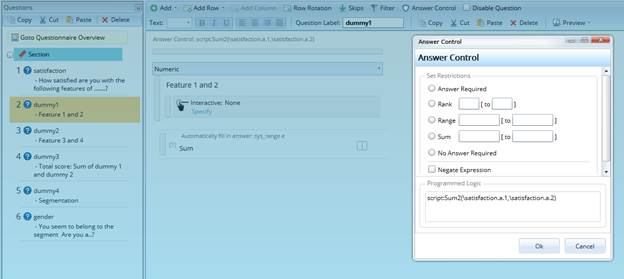In a preview question dummy1 looks like this: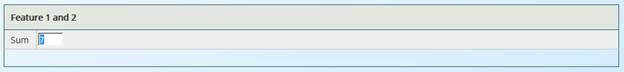Accordingly the dummy2 question is defined the same way: sum2(\satisfaction.a.3,\satisfaction.a.4)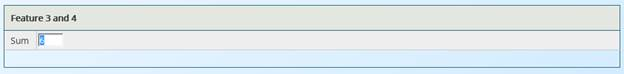Finally the total score is calculated in question dummy3 being the sum of questions dummy1 and dummy2. Sum2(\dummy1.a.1,\dummy2.a.1)

For the segment belonging we use the following conditional answer control:

1 when \dummy3.a.1=19:20 2 when \dummy3.a.1=15:18 3 when \dummy3.a.1=10:14 else 4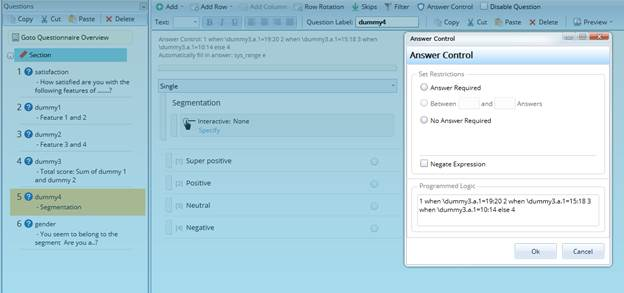Important: Note that the autofill attribute for a single and multiple question is vertically, i.e. it is set in the sub-question attributes. In numeric, open and in grids the attribute is set in the row(s).

So this answer control script will then calculate the only valid answer to be category 3 (Score is 13) hence the autofill question = sys_range e will fill in category 3 automatically.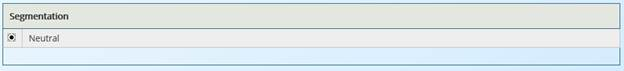In the next question, you may now address the result from question dummy4 as normal, e.g. used in a reference to question element: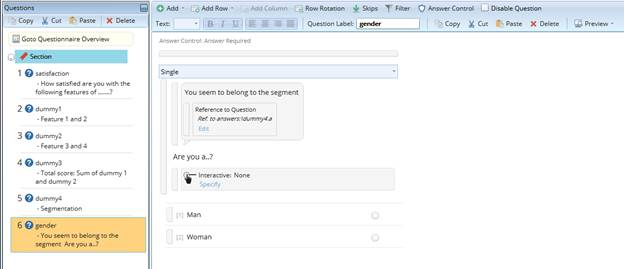Shown in the web it looks as this: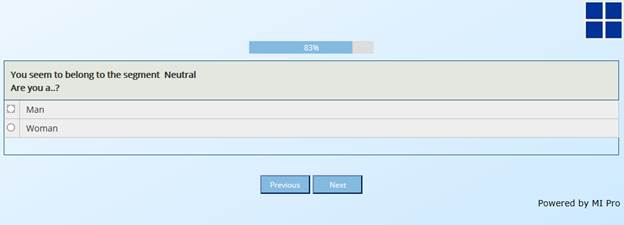3. Making the questions hidden
In all the autofill syntaxes the last letter has been "e". This will show you that the result is correct, and is beat practice when scripting the questionnaire.

If you want these dummy questions to be hidden, you simply change the letter "e" with a "c", and all questions from dummy1 to dummy4 will be hidden.